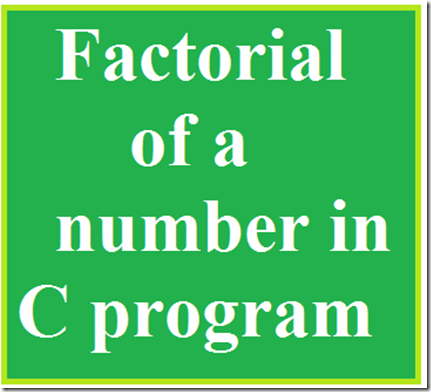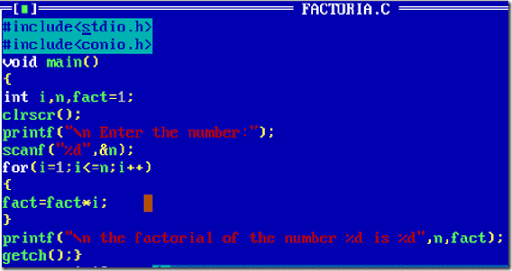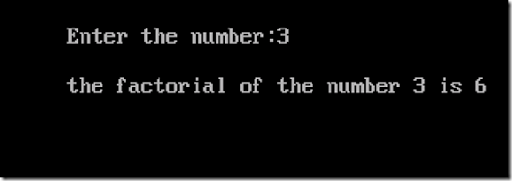# How to find Factorial of a number using C programFactorial of a number is nothing but the multiplies of every number in a specified number .
For example the factorial of the number 2 may be 1*2=2 , for 3 1*2*3=6.
It is easy to find the factorial of an number easily using c programming.
#include<stdio.h>
#include<conio.h>
Void main()
{
int i,n,fact;
clrscr();
printf(“\n Enter the number:”);
scanf(“%d”,&n);
for(i=1;i<=n;i++)
{
fact=fact*i;
}
printf(“\n the factorial of number %d is %d”,n,fact);
getch();
}Then after typing the above program in a compiler then compile it and run it and the output will be displayedProgram Explanation:
In this program the first two lines represent the header files and then the void main() function from which the actual execution starts.
Then i have initialised three variables i , n and fact.
‘ n ‘ this variable i have used to get the input from the user, ‘ fact ’ is the variable used to store the factorial value that we needed and the variable ‘ i ’ is used to execute the for loop.
for(i=1;i<=n;i++)
{
fact=fact*i;
}
in this at first the fact=1 and i=1 is initialised  then the loop is repeated till the user input n .
at first time the loop executed
fact=fact*i that means
fact=1*1 so fact=1
then the i value is incremented and the condition is checked with the user’s input in this program the user’s input is 3.
So the loop is repeated till the i value becomes 3.
So for the next time the fact value will be
fact=2*1=2
and for the next time
fact=2*3=6
so the factorial of the number 3 is 6.
Thus we have simply calculated the factorial value of a number using c programming.
If you are confused in understanding the for loop concept then read this tutorial
Loop Controlled instructions.